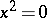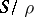# Locally finite semi-group

(diff) ← Older revision | Latest revision (diff) | Newer revision → (diff)
A semi-group in which every finitely-generated sub-semi-group is finite. A locally finite semi-group is a periodic semi-group (torsion semi-group). The converse is false: There are even torsion groups that are not locally finite (see Burnside problem). Long before the solution of the Burnside problem for groups, examples had been constructed of semi-groups that are torsion but not locally finite in classes of semi-groups remote from groups; above all, in the class of nil semi-groups (cf. Nil semi-group). These are, for example, a free semi-group with two generators in the variety given by, and a free semi-group with three generators in the variety given by. Moreover, for a number of classes of semi-groups the conditions of periodicity and local finiteness are equivalent. A trivial example is given by commutative semi-groups. A band of locally finite semi-groups (see Band of semi-groups) is itself a locally finite semi-group ; moreover, a semi-group that has a decomposition into locally finite groups is a locally finite semi-group; in particular, a semi-group of idempotents (cf. Idempotents, semi-group of) is a locally finite semi-group . Ifis such that any group satisfying the lawis locally finite, then any semi-group with the lawis locally finite . A semi-group that has a decomposition into locally finite semi-groups need not be a locally finite semi-group , but ifis a congruence on a semi-groupsuch that the quotient semi-groupis locally finite and every-class that is a sub-semi-group is locally finite, thenis a locally finite semi-group (see , ); in particular, an ideal extension of a locally finite semi-group by a locally finite semi-group is itself a locally finite semi-group. Ifis a periodic semi-group of matrices over a skew-field and all subgroups ofare locally finite, thenis locally finite , which implies that any periodic semi-group of matrices over an arbitrary field is locally finite.
Ifis a periodic inverse semi-group of matrices over a field and, moreover, the periods of all its elements (see Monogenic semi-group) are uniformly bounded and are not divided by the characteristic of the field, thenis finite .